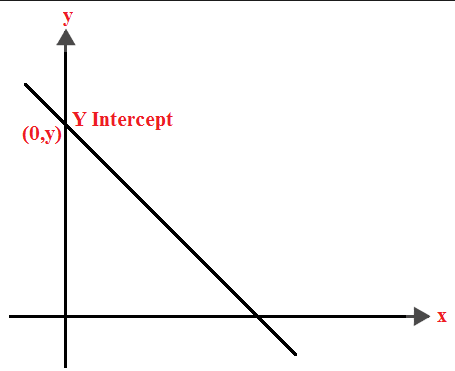Q

# Need clarity, kindly explain! - If the line intersects the x-axis at the point A and the y-axis at the point B,then the incentre of - Co-ordinate geometry - JEE Main

If the line intersects the x-axis at the point A and the y-axis at the point B,then the incentre of the triangle OAB, where O is the origin, is:

• Option 1)

(3,4)

• Option 2)

(2,2)

• Option 3)

(4,3)

• Option 4)

(4,4)

Views

y-intercept -

The distance on the y-axis from the origin where the straight line cuts it.

- whereinFrom the fig (r,r) is the center of circle.

Distance from point (r,r) to the line

centre is (2,2)

Option 1)

(3,4)

Option 2)

(2,2)

Option 3)

(4,3)

Option 4)

(4,4)

Exams
Articles
Questions